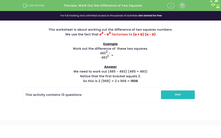# Work Out the Difference of Two Squares

In this worksheet, students will calculate the difference of two squares.Key stage:  KS 3

Curriculum topic:   Number

Curriculum subtopic:   Understand Integer Powers/Real Roots

Difficulty level:#### Worksheet Overview

This activity is about working out the difference of two square numbers.

We use the fact that a2 - b2 factorises to (a + b) (a - b)

Example

Work out the difference of  these two squares:

4852 - 4832

We need to work out (485 + 483) (485 - 483)

Notice that the first bracket equals 968 and that the second bracket equals 2

So this is 968 x 2 = 1,936

Being able to find the difference between two squares in this way can sometimes make it possible to do the calculation without the use of a calculator!

Let's have a go at some questions.

### What is EdPlace?

We're your National Curriculum aligned online education content provider helping each child succeed in English, maths and science from year 1 to GCSE. With an EdPlace account you’ll be able to track and measure progress, helping each child achieve their best. We build confidence and attainment by personalising each child’s learning at a level that suits them.

Get started